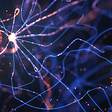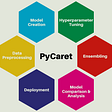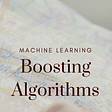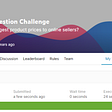# Best Books to Learn Machine Learning Algorithms

Machine learning algorithms are a series of steps used to find the relationship between labels and features of a dataset. Machine learning algorithms play an important role in building applications and models based on machine learning. There are so many machine learning algorithms that if you want to learn each of them in detail, you have to follow a book. So, in this article, I am going to introduce you to some of the best books for learning machine learning algorithms.

# Best Books to Learn Machine Learning Algorithms

When learning machine learning algorithms, you should focus on both its theory and its implementation using a programming language (most probably Python). Understanding the theory of machine learning algorithms will help you understand which algorithm should be used in what kind of problem, and understanding the implementation will help you train machine learning models, which is your end goal of learning machine learning algorithms. No book can teach you the complete theory as well as the implementation of machine learning algorithms at the same time.

So below are the two best books to learn machine learning algorithms where one book is completely focused on teaching you about the theory and mathematics behind machine learning algorithms, and the other book is focused on teaching you the implementation of machine learning algorithms using Python.

## Understanding Machine Learning: From Theory to Algorithms

This book focuses on the theory of machine learning algorithms. It also covers the math behind machine learning algorithms. While it does not cover all machine learning algorithms, you will find all of the commonly used and most important algorithms in this book. It’s easy to understand if you’re new to machine learning, but you need to have some basic knowledge of math to understand the math of machine learning algorithms. If you are familiar with the implementation of machine learning algorithms but have no knowledge of the theory of machine learning algorithms, you will face difficulties in choosing the best algorithm for a problem. Learning the theory of machine learning algorithms will also help you a lot in your job interviews. You can find this book here.

## Machine Learning Algorithms by Giuseppe Bonaccorso

This book is one of the best resources for learning the implementation of machine learning algorithms using the Python programming language. Although it also covers the theory of machine learning algorithms, but not as perfect as in the book mentioned above. You should only prefer this book if you are familiar with the theory of machine learning algorithms and want to learn how to implement them using Python. It covers all the machine learning algorithms you need to learn as a data scientist or machine learning engineer. One thing you should know is that this book is not for absolute beginners, you should know the basics of Python and Python libraries like NumPy, Pandas, Matplotlib, and Scikit-learn before you start with this book. You can find this book here.

# Summary

So these are the best books you can follow to learn the theory and implementation of machine learning algorithms. If you want to learn the theory of machine learning algorithms, you should choose “Understanding Machine Learning: From Theory to Algorithms”, and if you want to learn how to implement machine learning algorithms using Python, you should choose “Machine Learning Algorithms”. Hope you liked this article on the best books for learning machine learning algorithms. Free to ask your valuable questions in the comments section below.

## More from Aman Kharwal

I write stories behind the data📈 | https://www.instagram.com/the.clever.programmer/

Love podcasts or audiobooks? Learn on the go with our new app.

## Lets get active with “Activation Functions”## PyCaret —Prepare your Machine Learning model in minutes## 🚀Boosting Algorithms in Machine Learning## Beginners guide for Restricted Boltzmann Machine## How Has MS Voxel Deep Network Managed to Improve 3D Objects Recognition Using Cloud Map Only## Logistic Regression: The Cost Function## A Step-by-Step Case Study on the Mercari Price Suggestion Challenge by Kaggle## Aman Kharwal

I write stories behind the data📈 | https://www.instagram.com/the.clever.programmer/

## Data Science Quick Start (5-steps)## 10 tips to boost your Kaggle journey## Andrew Ng’s Machine Learning Simplified — Part 1## IPL Score Prediction Using Machine Learning